How Planes Fly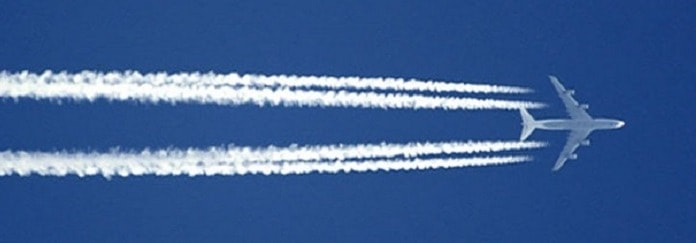Do you know how the planes fly? The physics of this process is the subject of our article. Of course, a plane is rather complex object and getting it in the air takes a bit of “rocket science” involved; thus, we should start with something simpler. Everybody knows how draft slaps doors. Sharp bangs happen when a fast flow of air goes through the door gap. This phenomenon is explained by Bernoulli’s principle, which is represented in liquids and gases. In elementary case of incompressible flow of true fluid, it looks as follows:

$$\frac{ \rho v^2}{2}+\rho gh+p=const$$

where $\rho$ is density, $v$ is speed, $g$ is acceleration of gravity, $h$ is level of flow (important in case of level change), аnd $p$ is pressure.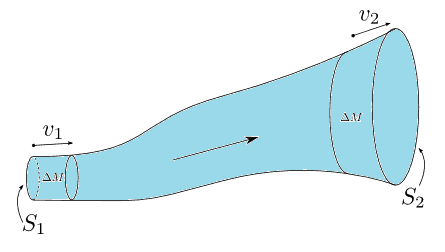The energy conservation law is the basis of Bernoulli’s law. The first two summands stand for the kinetic and potential energy per unit of volume. Being used for gases, formula is modified: in front of pressure $p$ there appears a constant coefficient depending on heat capacity ratio. In case of draft, the second summand becomes unchangeable. However, as speed of air flow rises, pressure goes down and there arises force that shuts the door. This phenomenon of hydrodynamics and gas dynamics, described by the Swiss physicist Daniel Bernoulli, plays obvious role in our life: various gas-, water-supply and aviation systems are calculated with its help.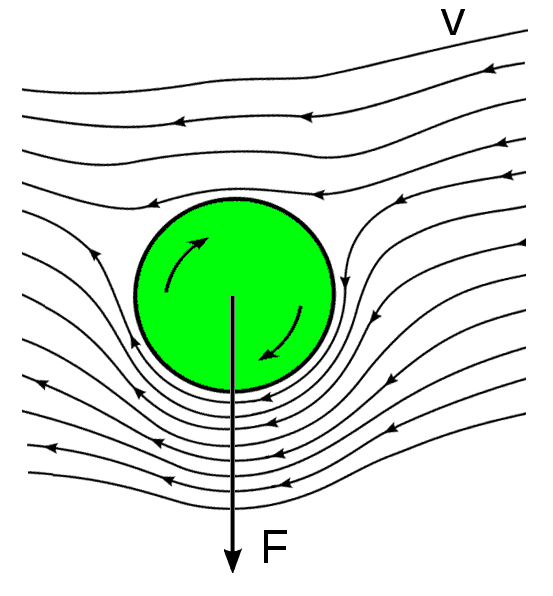Baseball, tennis and other ball players are familiar with the consequence of the Bernoulli’s principle – Magnus effect. One of the ways to kick the ball is called topspin which stands for spin about a horizontal axis perpendicular to the direction of travel, where the top surface of the ball is moving forward the spin. Quick spinning ball carries boundary level of air. As a result, speed of air flows at different sides of the ball appears to be different as well. Similar to Bernoulli’s principle, pressure is unequal and the ball falls under its influence. Ok, you may say, that’s great, but how the planes actually fly?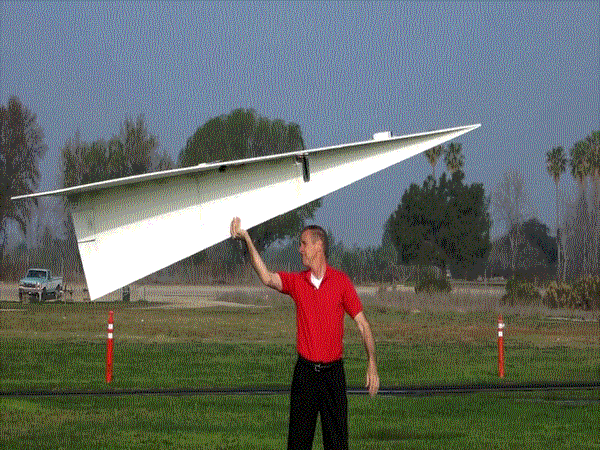Flight of the airplane is preconditioned be the geometry of its wing, and Bernoulli’s effect plays additional role in formation of lift. Modern planes can completely change geometry and aerofoil to optimize the flight at different speed, different modes of start and landing. Thus, in this article, we’ll cover only the simplest case with speed less than speed of sound. Wing has curved drop-shaped airfoil. While moving forward, air is being cut into two flows which have to pass over and under the wing within the same time. As the upper part of the wind is more protuberant than the lower, the upper flow has to pass with the faster speed. It provokes the pressure difference and the corresponding lift component. In the airplane photos from airshows, we can observe upper part of the wing wrapped into a white cloud. It results from condensation of water drops from the air at the area of low pressure over the wing during the maneuvers. Adiabatically extending towards the low pressure area, the air gets cooler, and its temperature can go below dew-point. Thus, the formation of drops appears. It is called Prandtl-Glauert singularity.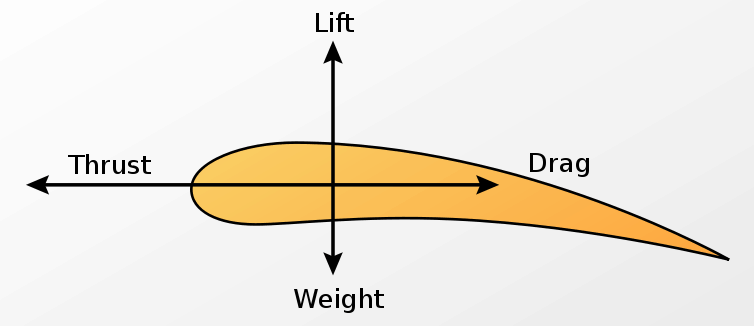The second component of the lift, which is basic in its contribution, appears from the angle of attack $\alpha$ (which is the angle between reference line on a body and the vector representing the relative motion between the body and the fluid through which it is moving). The air has mass and impulse, which it transfers to the wing. There appears additional force moving up and backwards. The first of two components contributes to the lift, while the second provides drag contribution. Numerical ratio between drag and lift depending on its aerofoil and angle of attack is called lift-to-drag ratio. In modern subsonic airplanes, it reaches 15, and in gliders – even 50.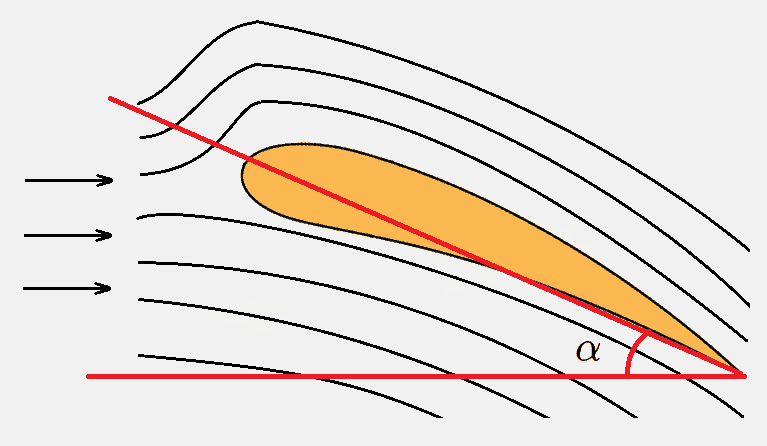Typical angle-of-attack point lies in the range 4-6 degrees, and pilots can regulate it during the flight. We can’t help mentioning that this interval is far from the point of stall angle of attack, at which lift reaches its maximum (usually 15-30 degrees). This owes to the fact that even small excess of the stall angle results in steep fall of the lift. It poses a threat of control failure of a flying machine.

Ok, now you probably have an idea about how planes fly. By the way, all this science stuff above was a joke. This is the true explanation:

1 Share
Filed under Engineering, Physics.